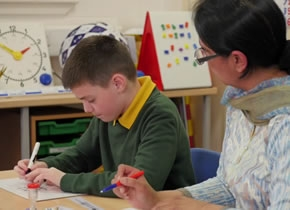### Find out moreRequest a full Catch Up® information brochure and details of our free information webinars

# News

### 1st November 2016 - Lynne’s logic – tackling subtractionHi everyone out there in Numberland, Lynne here again and looking today at that potential minefield which is subtraction.

Some points to remember first:

• As for addition, translate a calculation between the concrete, the oral and the symbolic representation. Relating a calculation to how it looks with practical apparatus, to an equivalent word problem and the written number sentence will help the child gain a deeper understanding of subtraction.

• Never make assumptions that the kiddiewinks are on the same wavelength as you. If you are talking about a ‘take away’, what is in their heads – are they thinking of a chicken tikka? I remember setting a problem for a child: “How many times can you subtract 7 from 43 and what is left afterwards?” Rather an unexpected answer: “I can subtract it as many times as I want and it leaves 36 every time.” A steep learning curve for me.

• There is no such thing as a subtraction sum. A subtraction calculation, yes, but sum is always addition.

• Children generally find counting back far more difficult than counting on. This is partly a result of the extra time spent counting on as opposed to counting back.

• You need to establish understanding of take away, and also an understanding of difference. As with all Catch Up® Numeracy, this should be done firstly through the numeracy activity, which will be essentially practical. The understanding gained can then be developed into the linked recording element. For older learners, number lines can be an important piece of the jigsaw.

With first steps, the numbers used should suit the concept involved, as will become clearer with the examples below.

• To develop early understanding, take away should be a counting back strategy, so the child should be asked to take away a small number, e.g. sixteen take away five = 16 – 5. It can be tricky for some children as once counting back has started, the first number has, to some extent, gone.

• If a number line or number track is used in the linked recording, the answer (11) will be found as one of the numbers on the number line or track.

• To develop early understanding of difference, use a counting on strategy, so the two numbers should be close together. For example: Find the difference between 12 and 17 = 17 – 12. Do not always say the larger number first, but the written calculation will put the larger number first. Using multilink cubes in the numeracy activity can be very useful. Make the two quantities into sticks which, when compared, show the difference clearly. Recording using two number lines one above the other, can support understanding in early sessions.

• If a number line is used to record a counting on strategy, the answer (5) is found by adding the jumps taken, which are usually recorded above the number line.

Once understanding of the concepts is embedded, then the strategy – counting on or counting back – should suit the numbers used, e.g. 53 – 45 by counting on, but 53 – 12 by counting back. To get to this level, however, will need lots of Catch Up Numeracy sessions for some learners. Beware of falling into the trap of always using only one strategy, whether it is counting on or counting back; this will lead to the learner trying to solve 56 – 47 by counting back, or trying to solve 56 – 17 by counting on.

Subtraction can be a bit of a minefield, but it is an important concept and should not be avoided. The secret is small steps which aim to develop understanding, rather than merely aiming to establish a process, and Catch Up® Numeracy addresses this requirement.